## Average Absolute Deviation

Average Absolute Deviation is summation of the absolute value of each deviation. It is useful to measure the spread of data while considering the effect of total number of data

Other name: average absolute error

Formula: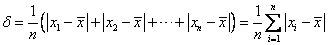Example: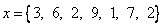You can try the interactive program of Mean Absolute Deviation (MAD) below. Simply click "Compute Mean Absolute Deviation" button.

To fill with your own input data, type your input data in the box below, separated by comma. Only numbers input are accepted. Then click Compute Mean Absolute Deviation button

Special for only two positive numbers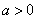and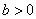, we have

Average Absolute deviation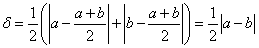Variance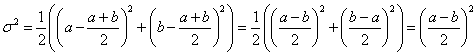If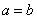, then variance and average absolute deviation which go to zero: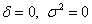,

If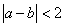, then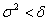If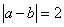, then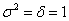If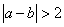, then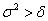Example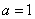,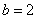, then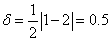Preferable reference for this tutorial is

Teknomo, Kardi (2015) Mean and Average. https:\\people.revoledu.com\kardi\ tutorial\BasicMath\Average\